• +91 9723535972
• info@interviewmaterial.com

# Syllogism Interview Questions and Answers

Question - 1 : -
In each of the following questions two statements are given and these statements are followed by two conclusions numbered (1) and (2). You have to take the given two statements to be true even if they seem to be at variance from commonly known facts. Read the conclusions and then decide which of the given conclusions logically follows from the two given statements, disregarding commonly known facts.

(A) If only (1) conclusion follows
(B) If only (2) conclusion follows
(C) If either (1) or (2) follows
(D) If neither (1) nor (2) follows and
(E) If both (1) and (2) follow.

Question
Statements: Some actors are singers. All the singers are dancers.

Conclusions:

Some actors are dancers.
No singer is actor.
A. Only (1) conclusion follows
B. Only (2) conclusion follows
C. Either (1) or (2) follows
D. Neither (1) nor (2) follows
E. Both (1) and (2) follow

Option A

Explanation: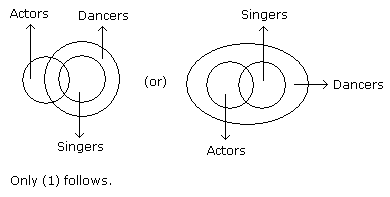Question - 2 : -
In each of the following questions two statements are given and these statements are followed by two conclusions numbered (1) and (2). You have to take the given two statements to be true even if they seem to be at variance from commonly known facts. Read the conclusions and then decide which of the given conclusions logically follows from the two given statements, disregarding commonly known facts.

(A) If only (1) conclusion follows
(B) If only (2) conclusion follows
(C) If either (1) or (2) follows
(D) If neither (1) nor (2) follows and
(E) If both (1) and (2) follow.

Question
Statements: All the harmoniums are instruments. All the instruments are flutes.

Conclusions:

All the flutes are instruments.
All the harmoniums are flutes.
A. Only (1) conclusion follows
B. Only (2) conclusion follows
C. Either (1) or (2) follows
D. Neither (1) nor (2) follows
E. Both (1) and (2) follow

Option B

Explanation: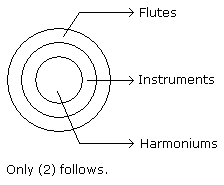Question - 3 : -
In each of the following questions two statements are given and these statements are followed by two conclusions numbered (1) and (2). You have to take the given two statements to be true even if they seem to be at variance from commonly known facts. Read the conclusions and then decide which of the given conclusions logically follows from the two given statements, disregarding commonly known facts.

(A) If only (1) conclusion follows
(B) If only (2) conclusion follows
(C) If either (1) or (2) follows
(D) If neither (1) nor (2) follows and
(E) If both (1) and (2) follow.

Question
Statements: Some mangoes are yellow. Some tixo are mangoes.

Conclusions:

Some mangoes are green.
Tixo is a yellow.
A. Only (1) conclusion follows
B. Only (2) conclusion follows
C. Either (1) or (2) follows
D. Neither (1) nor (2) follows
E. Both (1) and (2) follow

Option D

Explanation: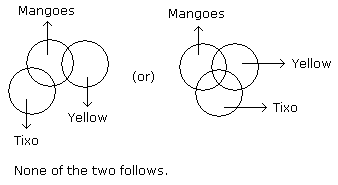Question - 4 : -
In each of the following questions two statements are given and these statements are followed by two conclusions numbered (1) and (2). You have to take the given two statements to be true even if they seem to be at variance from commonly known facts. Read the conclusions and then decide which of the given conclusions logically follows from the two given statements, disregarding commonly known facts.

(A) If only (1) conclusion follows
(B) If only (2) conclusion follows
(C) If either (1) or (2) follows
(D) If neither (1) nor (2) follows and
(E) If both (1) and (2) follow.

Question
Statements: Some ants are parrots. All the parrots are apples.

Conclusions:

All the apples are parrots.
Some ants are apples.
A. Only (1) conclusion follows
B. Only (2) conclusion follows
C. Either (1) or (2) follows
D. Neither (1) nor (2) follows
E. Both (1) and (2) follow

Option B

Explanation: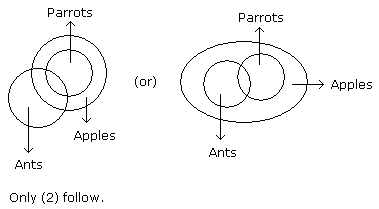Question - 5 : -
In each of the following questions two statements are given and these statements are followed by two conclusions numbered (1) and (2). You have to take the given two statements to be true even if they seem to be at variance from commonly known facts. Read the conclusions and then decide which of the given conclusions logically follows from the two given statements, disregarding commonly known facts.

(A) If only (1) conclusion follows
(B) If only (2) conclusion follows
(C) If either (1) or (2) follows
(D) If neither (1) nor (2) follows and
(E) If both (1) and (2) follow.

Question
Statements: Some papers are pens. All the pencils are pens.

Conclusions:

Some pens are pencils.
Some pens are papers.
A. Only (1) conclusion follows
B. Only (2) conclusion follows
C. Either (1) or (2) follows
D. Neither (1) nor (2) follows
E. Both (1) and (2) follow

Option E

Explanation:Question - 6 : -
In each of the following questions two statements are given and these statements are followed by two conclusions numbered (1) and (2). You have to take the given two statements to be true even if they seem to be at variance from commonly known facts. Read the conclusions and then decide which of the given conclusions logically follows from the two given statements, disregarding commonly known facts.

(A) If only (1) conclusion follows
(B) If only (2) conclusion follows
(C) If either (1) or (2) follows
(D) If neither (1) nor (2) follows and
(E) If both (1) and (2) follow.

Question
Statements: Some dogs are bats. Some bats are cats.

Conclusions:

Some dogs are cats.
Some cats are dogs.
A. Only (1) conclusion follows
B. Only (2) conclusion follows
C. Either (1) or (2) follows
D. Neither (1) nor (2) follows
E. Both (1) and (2) follow

Option D

Explanation: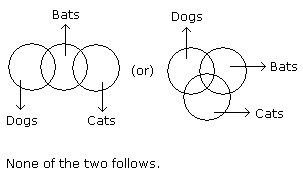Question - 7 : -
In each of the following questions two statements are given and these statements are followed by two conclusions numbered (1) and (2). You have to take the given two statements to be true even if they seem to be at variance from commonly known facts. Read the conclusions and then decide which of the given conclusions logically follows from the two given statements, disregarding commonly known facts.

(A) If only (1) conclusion follows
(B) If only (2) conclusion follows
(C) If either (1) or (2) follows
(D) If neither (1) nor (2) follows and
(E) If both (1) and (2) follow.

Question
Statements: All the trucks are flies. Some scooters are flies.

Conclusions:

All the trucks are scooters.
Some scooters are trucks.
A. Only (1) conclusion follows
B. Only (2) conclusion follows
C. Either (1) or (2) follows
D. Neither (1) nor (2) follows
E. Both (1) and (2) follow

Option D

Explanation: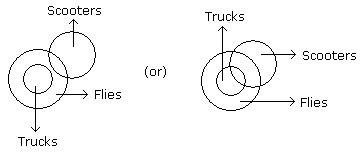Neither (1) nor (2) follows.

Question - 8 : -
In each of the following questions two statements are given and these statements are followed by two conclusions numbered (1) and (2). You have to take the given two statements to be true even if they seem to be at variance from commonly known facts. Read the conclusions and then decide which of the given conclusions logically follows from the two given statements, disregarding commonly known facts.

(A) If only (1) conclusion follows
(B) If only (2) conclusion follows
(C) If either (1) or (2) follows
(D) If neither (1) nor (2) follows and
(E) If both (1) and (2) follow.

Question
Statements: All buildings are chalks. No chalk is toffee.

Conclusions:

No building is toffee
All chalks are buildings.
A. Only (1) conclusion follows
B. Only (2) conclusion follows
C. Either (1) or (2) follows
D. Neither (1) nor (2) follows
E. Both (1) and (2) follow

Option A

Explanation: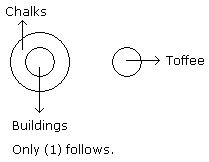Question - 9 : -
In each of the following questions two statements are given and these statements are followed by two conclusions numbered (1) and (2). You have to take the given two statements to be true even if they seem to be at variance from commonly known facts. Read the conclusions and then decide which of the given conclusions logically follows from the two given statements, disregarding commonly known facts.

(A) If only (1) conclusion follows
(B) If only (2) conclusion follows
(C) If either (1) or (2) follows
(D) If neither (1) nor (2) follows and
(E) If both (1) and (2) follow.

Question
Statements: All cars are cats. All fans are cats.

Conclusions:

All cars are fans.
Some fans are cars.
A. Only (1) conclusion follows
B. Only (2) conclusion follows
C. Either (1) or (2) follows
D. Neither (1) nor (2) follows
E. Both (1) and (2) follow

Option D

Explanation: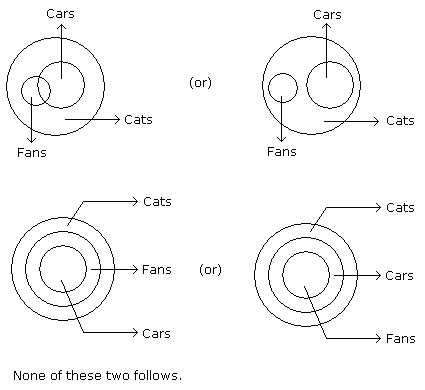Question - 10 : -
In each of the following questions two statements are given and these statements are followed by two conclusions numbered (1) and (2). You have to take the given two statements to be true even if they seem to be at variance from commonly known facts. Read the conclusions and then decide which of the given conclusions logically follows from the two given statements, disregarding commonly known facts.

(A) If only (1) conclusion follows
(B) If only (2) conclusion follows
(C) If either (1) or (2) follows
(D) If neither (1) nor (2) follows and
(E) If both (1) and (2) follow.

Question
Statements: All the actors are girls. All the girls are beautiful.

Conclusions:

All the actors are beautiful.
Some girls are actors.
A. Only (1) conclusion follows
B. Only (2) conclusion follows
C. Either (1) or (2) follows
D. Neither (1) nor (2) follows
E. Both (1) and (2) follow

Option E

Explanation: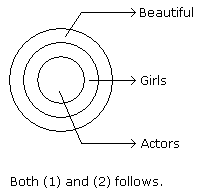Name:
Email:

# Latest News# 10000+ interview questions in different categories